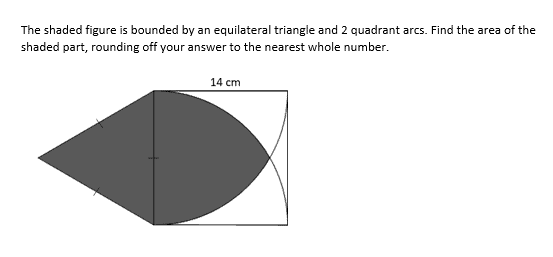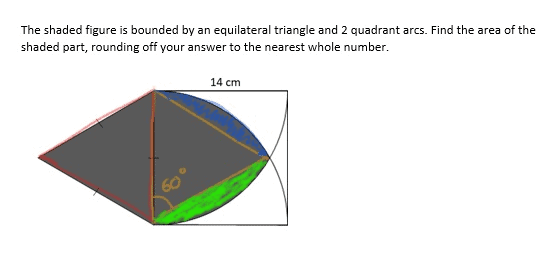# QuestionHi can anyone help me with this question? Thank youThis is a ‘cut and paste’ question, where you will have to split the shaded area into portions.

As we can see, the orange equilateral triangle can be reflected nicely onto the right side, into the square. This is possible because the two yellow lines are also the radii of the quadrants, and will have the same length as the sides of the triangles.

If we combine the blue portion with the yellow equilateral triangle, it will give us part of circle, and we can see that it is 60°. In order to calculate this part, we take (60/360) x (22/7) x (14) x (14) which will give us 308/3.

We multiply the above answer by 2, as we can see that the green portion can be combined with the orange triangle to give the same area.

We round off the answer to 205.

Hope this helps! Do let me know if you need more clarifications and feel free to PM me.

http://www.futureachievers.com.sg

9114 3397